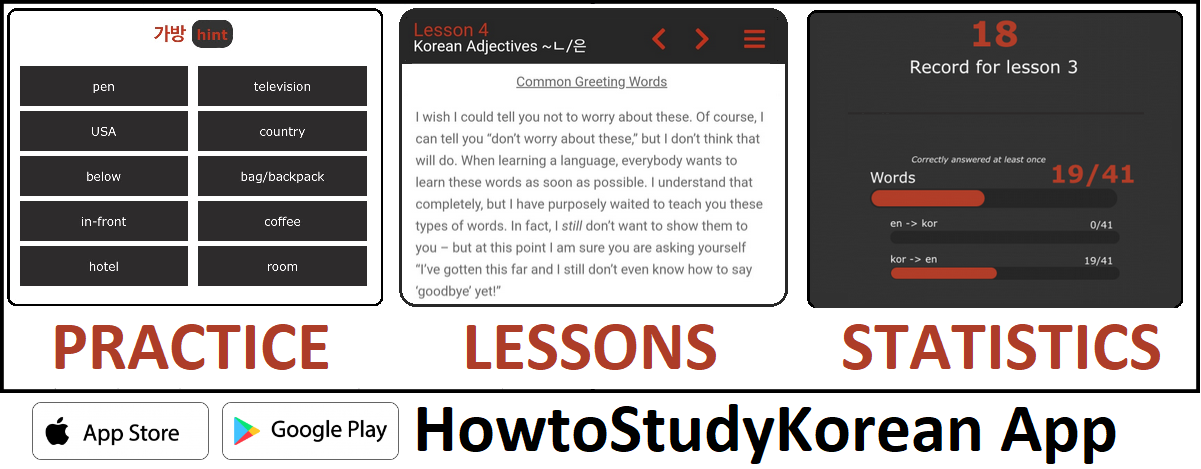Also available in: in: Türkçe, کوردی ,العربية

Forget how to read? Don’t worry! Take a look at the letters bellow and get them back into your memory!

ㅂ= b
ㅈ= j
ㄷ= d
ㄱ= k
ㅅ= s
ㅁ= m
ㄴ= n
ㅎ= h
ㄹ= r/l
ㅇ = silent letter or -ng

ㅣ= i
ㅏ= a
ㅓ= Romanized as “eo” but it sounds closer to “uh” in English
ㅡ= eu
ㅜ= u
ㅗ= o

ㄲ = kk
ㅋ = k

ㅃ = bb
ㅍ = p

ㅉ = jj
ㅊ = ch

ㄸ = dd
ㅌ = t

ㅆ = ss

ㅑ = ya
ㅕ = yeo
ㅠ = yu
ㅛ = yo
ㅐ = ae  (identical to ㅔ)
ㅔ = e (identical to ㅐ)
ㅟ = wi
ㅝ = wo
ㅚ = oe
ㅘ = wa
ㅢ = ui

ㅙ = wae
ㅒ= yae
ㅖ = ye
ㅞ = we

Ok, I got it! Take me back to the Unit 1 Homepage!